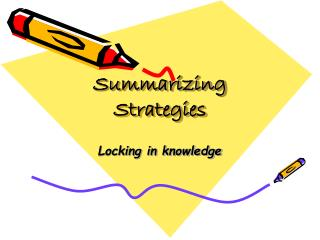DownloadDownload PresentationSummarizing Strategies

# Summarizing Strategies

Download Presentation## Summarizing Strategies

- - - - - - - - - - - - - - - - - - - - - - - - - - - E N D - - - - - - - - - - - - - - - - - - - - - - - - - - -
##### Presentation Transcript

1. Summarizing Strategies Locking in knowledge

2. Top 5 LFS/Marzano Strategies

3. Summarizing: • Student Review • Student Elaboration • Student Guided Practice • Student summarization is a learning strategy not a teaching strategy. • Distributed throughout a lesson - not just at the end

4. To be effective the student summarizes what they have learned. This is not the same as the teacher summarizing what they have taught!

5. Key Points - • All students participate • Allocate time – do not skip to “catch up” • Use feedback during summarizing to monitor student understanding • Frequent summarizing prevents misconceptions • Focuses on a key point of the lesson

6. Distributed Summarizing Strategies • Pair Share • Draw a picture • Guided practice with summarizing statements • Statement of understanding

7. Summarizing at the end of the lesson • Should answer the essential question • Can be informal or formal • Causes students to create a schema/ context for new knowledge and skills • Provides the teacher with information on skills that need to be re-taught

8. CulminatingSummarizing Strategies • The most important thing • 3 – 2 – 1 • Reflection Activities • Letter to absent student • End of lesson ticket • Culminating strategies should verify that students can answer the lesson essential question

9. Essential Question Design • Should be written in “student language” • Should include the skills and or vocabulary required to answer the question correctly • Lesson EQs should be more specific than unit EQs • Students should know what the EQ for each lesson and unit is before they start. • Refer back to unit EQs as students get pieces of the answer • The next EQ should build on the answers of prior questions and lead to the unit EQ

10. Nuts and bolts of writing EQs • Can you turn your objective/standard into an effective question? • What question would you ask at the end of a lesson to see if the students “get it” before moving on? • What questions will foster transfer? • Make sure: • The question does NOT have a yes/no answer • The question connects skills and content • Multiple part questions don’t need to be split into 2 separate questions. • KEEP IT SIMPLE

11. What was Lincoln’s purpose in his 2nd inaugural address? How should I define the process of mitosis? How does knowing how to make inferences help me identify Lincoln’s purpose in his 2nd inaugural? How can I use sequencing to help me define and explain mitosis based on the assigned article? Sample Skill-based EQs

12. How do you solve complex single variable equations? What are 3 causes of the spread of AIDS? How would I use the associative and distributive properties to solve complex single variable equations? How does understanding cause and effect help me understand the spread of AIDS? More EQs